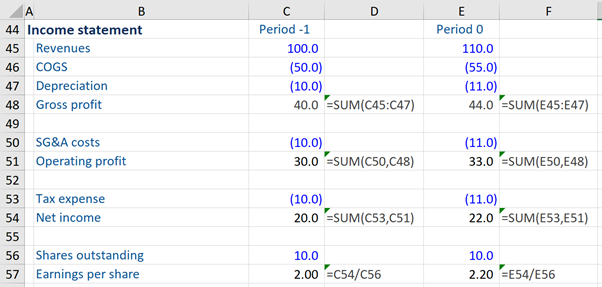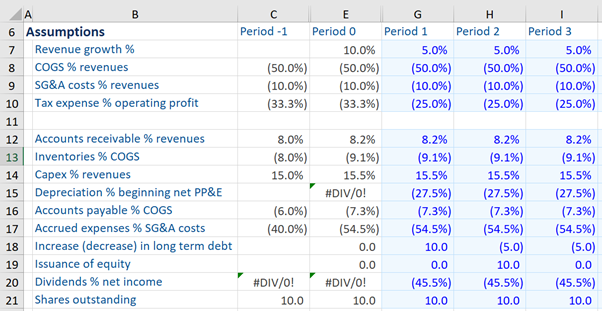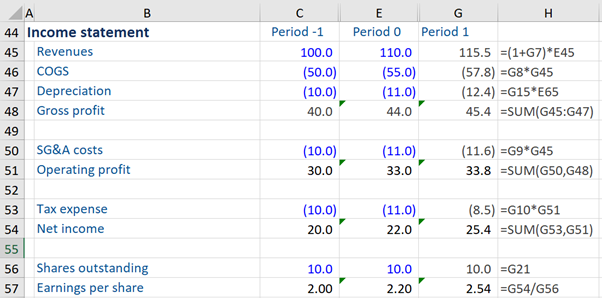## What is “Forecasting Income Statement Line Items”?

As part of the financial modeling process, analysts will need to forecast the company’s income statement. This is typically done with ‘line-by-line’ once assumptions have been made for future growth. Put simply, the analyst works through its line on the income statement (starting with revenues) and provides an estimate for future performance using a series of assumptions. Here is a reminder of the four steps to build a financial model

1.      Input the company’s publicly available (or private) historical data – a financial model’s projections are typically based on the historical financial performance

2.      Construct ratios (such as COGS as % of sales) and statistics predicated on the historical data

3.      Making assumptions based on the above two steps

4.      Forecast the income statement line items using these assumptions

Usually, the forecasting begins with the income statement and then continues into the balance sheet and cash flow statement. Once the majority of the forecasts are made, the 3 statements can be linked up. The income statement is a very useful forecasting tool as it can help analyze the operating capabilities of a company and provide close-up analysis of the sales potential along with likely upcoming costs.

## Key Learning Points

• To facilitate company analysis and valuation, investors often need to forecast future sales and earnings from the company’s income statement
• The assumptions made by an analyst about future business conditions drive the forecasts – this is the basis of building a financial model
• A good modeler, when attempting to forecast the line items of an income statement, should initially build the forecast of the same for the first forecast period only (i.e. period 1) and check it thoroughly before copying it across to the later forecast years (i.e. period 2 and beyond)
• To forecast the income statement line items, one should, at the outset, fill up some subtotals in the income statement for the historical period (i.e. period -1)

## Assumptions, Checking and Copying Across the Forecast Period

When forecasting line items it is helpful to have a set process to follow to avoid confusion – usually, this can help avoid both duplication or forgotten lines, plus it can help avoid unwanted circular links which can be very unhelpful!

### Look to historical data, such as growth rates, when making assumptions

The first key point to note is that assumptions play a pivotal or central role in forecasting the line items of the income statement. For example, if historical sales have gone up 10% every historical year, this assumption can continue into the projection period. Thus, based on assumptions, if year 0 (historical period) sales were \$100, then this year’s (i.e. first forecast period) sales would be \$110 if applying a 10% growth rate.

### Complete Period 1 before copying into future years

Secondly, when forecasting the income statement it is best to complete all the lines of a full year of forecasts (e.g. year 1) before copying the assumptions over to future years.

Year 1 should be built and checked thoroughly, before copying across, to later (forecast) years. A good modeler will resist the urge to copy across each line as it is built. It is best to build a section of the model and check it thoroughly before copying across. This tends to be the best way to avoid errors.

This method is more efficient time-wise but more importantly, is likely to be more accurate also. Occasionally, each line must be copied across individually, but copytable formulas are much more commonly used.

### Add subtotals, such as Gross Profit, to historical periods and ALWAYS copy over to avoid errors

Lastly, the subtotals are calculated in the historical period (using historical data) and are known to be correctly built. Therefore, these subtotals should be copied across and not rebuilt.

## Example – Forecasting Income Statement – Line Items

In the example below, we build a forecast income statement line items for only the first forecast period. Let’s start by having a quick look at the income statement. Here we see that we already have historical data for period -1 and period 0.If we look at the second workout below, we can view some ratios and statistics based on the historical figures given in the first workout. We can also see some forecast assumptions given (based on the historical figures and ratios).

### Forecasting Revenue and Cost assumptions (to Gross profit)

In our example, revenue growth assumption is 5% in period 1 (i.e. the first forecast period). Likewise, the COGS % of revenues and SG&A costs % revenues assumptions for period 1 are given. These assumptions are given for period 2 and 3 too, but we will ignore that for the time being.Second, we total the operating profit for period -1, which is the sum of gross profit and SG&A costs. Third, we total the net income, which is the sum of operating profit and tax expense.

Finally, we copy each of these subtotals into period 0 (historical period) and also period 1 (first year of the forecast). This will be helpful for checking that the assumptions are translating into realistic and sensible sales and profit forecasts.

All these calculations are shown in the first workout above.

### Forecasting period 1

Having done the above, we now will start forecasting period 1 (i.e. first forecast period).

Looking at the assumptions at the top, we know that revenue growth is forecast to be 5% for the next two upcoming years. So we must add a formula for period 1, based on the revenue growth assumption of 5%. By taking the 110 in Period 0 and multiplying 110 by (1 + 0.05) which equals 115.5 we can get a revenue forecast for Period 1 based on our assumptions.Next, we move on to forecast COGS. Again we refer to the assumptions in the workout above and we see that the COGS as a % of revenues assumption for period 1 is 50%. So, to get the COGS forecast for period 1 (forecast period), we multiply 50% by revenue of the last year (historical period) of 110 to arrive at the figure (57.8). You can refer to the formula in the table for more detail.

Next, is forecasting depreciation which again refers to the assumptions in the workout above. The interesting point here is that the depreciation assumption for period 1 is 27.5% of the beginning net PP&E – which is the same thing as the net PP&E for the last year (historical period) that is found in the balance sheet (not shown here) and is 45.0. So, to get the depreciation forecast for period 1, we multiply 27.5% and net PP&E of last year i.e. 45 and we get the depreciation forecast of (12.4) for period 1.

Next, we find that the gross profit forecast for period 1 is already done now by summing the forecasts of revenues, cogs, and depreciation to arrive at the figure of 45.4.

We use the same process for SG&A costs – to obtain the SG&A forecasts for period 1, we multiply this assumption by the forecast revenue (period 1), which is 115.5, and obtain the figure of (11.6). Further, operating profit is now done too (33.8) by summing SG&A costs and gross profit.

The last line item is the tax expense. Here our tax expense % operating profit assumption for period 1 is 25%. Now, to get the tax expense forecast for period 1, we simply multiply this assumption by an operating profit forecast of 33.8 to get a period 1 tax expense forecast of (8.5). So, the net income forecast for period 1 is 25.4. Tax expense needs to be deducted from operating profit to arrive at the net income forecast.

Earnings per share is calculated as net income divided by the number of shares outstanding. We do this calculation for the first historical period first (i.e. period -1) and then copy it through to period 0. Then for period 1, we calculate the EPS for period 1 by taking the share outstanding assumption of 10% for period 1 (forecast period) and thereafter, dividing forecast net income for period 1 by the shares outstanding for period 1 to arrive at the figure of 2.54 for this forecast period. If there are any share buybacks planned or new shares issued this ought to be reflected in the number of shares on the income statement.

## Conclusion

By sticking to the careful process and completing the first period fully before copying over, we can make sure the income statement forecasts are completed carefully and accurately.

Once familiar with this process it is a relatively simple (but regimented) process to come out with income statement forecasts. Sometimes the income statement layout will be altered to accommodate the details provided by a company – perhaps there will be an additional line of detail as R&D costs are broken out or more cost detail provided. However, the same principles remain in place for each income statement.